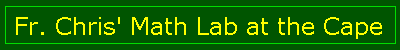Main Page

Lessons
Angles
Pool
Oil Pump
Basic Triangles
More Triangles
Art
Web Page
Euclid
Homework
Geometry
Hon Geometry

Web Calc
Pythagorean
Square Root
Fractions

Trivia
Impossibles
Trivia Quiz
Optical Quiz

TI Calc
Astronomy
Capuchins
FAQ

E-mail me!

# Questions

Note well:

The values for ray height should be betwen 0 and 1. This

The wavelength should between 400 nanometers and 700 nm.

1. What is the wavelength for green?
2. What is the wavelength for blue?
3. What is the wavelength for violet?
4. What is the wavelength for red?
5. What is the wavelength for orange?
6. Does each color bend the same? Why or Why not?
7. If the angle inside the raindrop is 47 degrees, how many degrees of arc is it between the entrance of light and the exit?
8. Look at http://www.geom.umn.edu/education/calc-init/rainbow/experiment.html Try typing these three sets of parameters in the first column:

### Set A

Send in 3 incoming beams of light at: 0.05, 0.1, 0.15

### Set B

Send in 3 incoming beams of light at: 0.5, 0.55, 0.6

### Set C

Send in 3 incoming beams of light at: 0.75, 0.8, 0.85

Sketch the results. Which set has the outgoing rays the most concentrated? The most diffuse?

9. For sets A, B and C, does it matter if you use the same colors or different ones?
10. Look at http://www.geom.umn.edu/education/calc-init/rainbow/conclusion.html. Why are the colors of a rainbow experienced as separate stripes of color and not all mixed together around each droplet? Draw a picture if it helps to explain yourself.
11. Which color is always on the top of a rainbow? Why?